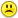+0

# Partial fractions

0
1352
9

Express the following as a partial fraction:

a) f(x)=2x-13/(2x+1)(x-3)

b)f(x)=x^2 +5x +7/(x+2)^3

c) f(x)= x^2 -10/ (x-2)(x+1)

Sorry dont know how to do any of these.

Nov 15, 2014

#7
+10

That just leaves one more

c)

$$\\f(x)=\frac{x^2-10}{(x-2)(x+1)}\\\\ f(x)=\frac{x^2-10}{x^2-x-1}\\\\$$

We need to do an algebraic (or synthetic) division

$$\begin{tabular}{ccccccc} &&&\;1&&& &&&&||&||&||& \\ x^2&-x&-1&\|\;x^2&0&-10\\ &&&\;x^2&-x&-1\\ &&&||&||&||&& &&&&+x&-9&\\ \end{tabular}$$

so

$$\\\frac{x^2-10}{(x-2)(x+1)}=1\;+\;\frac{x-9}{x^2-x-1}\\\\ \frac{x^2-10}{(x-2)(x+1)}=1\;+\;\frac{x-9}{(x-2)(x+1)}\\$$

Now let

$$\\\frac{x-9}{(x-2)(x+1)}=\frac{A}{x-2}+\frac{B}{x+1}\\\\ \frac{x-9}{(x-2)(x+1)}=\frac{A(x+1)+B(x-2)}{(x-2)(x+1)}\\\\$$

Equating numerators we have

$$\\x-9=A(x+1)+B(x-2)\\\\ x-9=(A+B)x+(A-2B)\\\\ so\\ A+B=1\quad\rightarrow\quad A=1-B\\ -9=A-2B\\ -9=1-B-2B\\ -10=-3B\\ B=10/3\\\\ A=1-10/3\\ A=1-3/3-7/3\\ A=-7/3$$

therefore

$$\\f(x)=\frac{x^2-10}{(x-2)(x+1)}\\\\ f(x)=1\;+\;\frac{x-9}{(x-2)(x+1)}\\\\ f(x)=1\;-\;\frac{7/3}{x-2}+\frac{10/3}{x+1}\\\\$$

Nov 16, 2014

#1
+5

a)

I haven't done these in ages. Let's see if I can remember.

There is probably a much simpler way of doing this but here goes

$$\\\frac{2x-13}{(2x+1)(x-3)}\\\\ =\frac{A}{2x+1}-\frac{B}{x-3}\\\\ =\frac{A(x-3)-B(2x+1)}{(2x+1)(x-3)}\\\\ =\frac{Ax-3A-2Bx-B}{(2x+1)(x-3)}\\\\ =\frac{(A-2B)x-(3A+B)}{(2x+1)(x-3)}\\\\ so\\\\ A-2B=2\qquad and \qquad 3A+B=13\\ A=2+2B\\ 3(2+2B)+B=13\\ 6+7B=13\\ 7B=7\\ B=1\\ A=2+2*1\\ A=4\\ so\\\\ \frac{2x-13}{(2x+1)(x-3)}=\frac{4}{2x+1}-\frac{1}{x-3}$$

Nov 15, 2014
#2
0

ahh thank you! that's perfect

Nov 15, 2014
#3
0

I am too tired to do more tonight - It is 1:30am.

The others are a little different I think.  If you google it you are bound to find a good you tube that will show you how to do the others.  There are lots of great maths you tubes and also other maths resource, that is how i often learn things. :)

Nov 15, 2014
#4
0

Oh ahaha it's 14.51 here! Okay, I'll look into it now. Thankyou!

Nov 15, 2014
#5
+10

b)f(x)=x^2 +5x +7/(x+2)^3 = A/(x+2) + B/(x + 2)^2 + C/(x + 2)^3 ....multiply both sides by (x+2)^3....so we have

x^2 + 5x + 7 =   A(x+2)^2 + B(x+2) + C

x^2 + 5x + 7 = A(x^2 + 4x + 4) +B(x + 2) + C

x^2 + 5x + 7 = A(x^2 + 4x + 4) +B(x + 2) + C

x^2 + 5x + 7 = Ax^2 +(4A+ B)x + (4A + 2B + C)

So, equating coefficients..

A = 1

4A + B = 5  →  4 + B = 5 →  B = 1

4A + 2B + C = 7  → 4 + 2 + C = 7 →  6 + C = 7   → C  = 1

So we have...

x^2 +5x +7/(x+2)^3  =  1/(x+2) + 1/(x + 2)^2 + 1/(x + 2)^3Nov 16, 2014
#6
+5

Ah Chris you beat me to it I was just about to present my answer.

so Part B is now officially solved.Nov 16, 2014
#7
+10

That just leaves one more

c)

$$\\f(x)=\frac{x^2-10}{(x-2)(x+1)}\\\\ f(x)=\frac{x^2-10}{x^2-x-1}\\\\$$

We need to do an algebraic (or synthetic) division

$$\begin{tabular}{ccccccc} &&&\;1&&& &&&&||&||&||& \\ x^2&-x&-1&\|\;x^2&0&-10\\ &&&\;x^2&-x&-1\\ &&&||&||&||&& &&&&+x&-9&\\ \end{tabular}$$

so

$$\\\frac{x^2-10}{(x-2)(x+1)}=1\;+\;\frac{x-9}{x^2-x-1}\\\\ \frac{x^2-10}{(x-2)(x+1)}=1\;+\;\frac{x-9}{(x-2)(x+1)}\\$$

Now let

$$\\\frac{x-9}{(x-2)(x+1)}=\frac{A}{x-2}+\frac{B}{x+1}\\\\ \frac{x-9}{(x-2)(x+1)}=\frac{A(x+1)+B(x-2)}{(x-2)(x+1)}\\\\$$

Equating numerators we have

$$\\x-9=A(x+1)+B(x-2)\\\\ x-9=(A+B)x+(A-2B)\\\\ so\\ A+B=1\quad\rightarrow\quad A=1-B\\ -9=A-2B\\ -9=1-B-2B\\ -10=-3B\\ B=10/3\\\\ A=1-10/3\\ A=1-3/3-7/3\\ A=-7/3$$

therefore

$$\\f(x)=\frac{x^2-10}{(x-2)(x+1)}\\\\ f(x)=1\;+\;\frac{x-9}{(x-2)(x+1)}\\\\ f(x)=1\;-\;\frac{7/3}{x-2}+\frac{10/3}{x+1}\\\\$$

Melody Nov 16, 2014
#8
0

Very nice, Melody.....I didn't know what to do when the powers on each polynomial in both numerator and denominator were equal....Thanks for that "refresher"....(although.....if I didn't know what to do.....apparently......there was nothing to "refresh"....!!!)Nov 16, 2014
#9
0

Thanks Chris,

Yes, there was a lot of 'refreshing' happening for me on these questions too.It is good to have a challenge once in a while and we both learned from itNov 17, 2014